用 Python 自學資料科學與機器學習入門實戰：Matplotlib 基礎入門什麼是 Matplotlib？

Python 的視覺化套件有靜態的 MatplotlibSeabornggplot（借鏡於 Rggplot2）套件以及動態的 Bokeh 套件（類似於 D3.js）。其中 Matplotlib 是 Python 的一個重要模組（Python 是一個高階語言也是一種膠水語言，可以透過整合其他低階語言同時擁有效能和高效率的開發），主要用於資料視覺化上。一般來說使用 Matplotlib 有兩種主要方式：直接和 Matplotlib 的全域 pyplot 模組互動操作，第二種則是物件導向形式的操作方式。若是只有一張圖的話使用全域 pyplot 很方便，若是有多張圖的話用物件導向操作。一般來說 Matplotlib 預設值並不理想，但它的優點在於很容易在上面外包一層提供更好的預設值或是自己修改預設值。

第一張 Matplotlib 圖片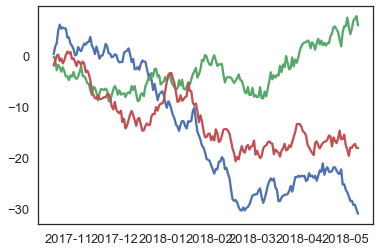物件導向式 Matplotlib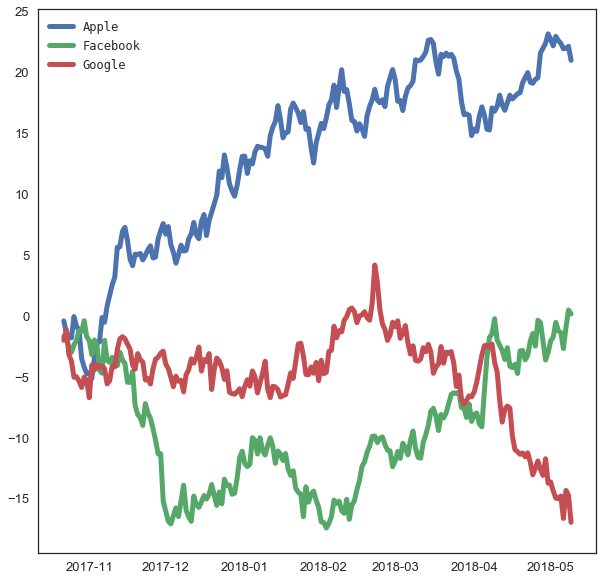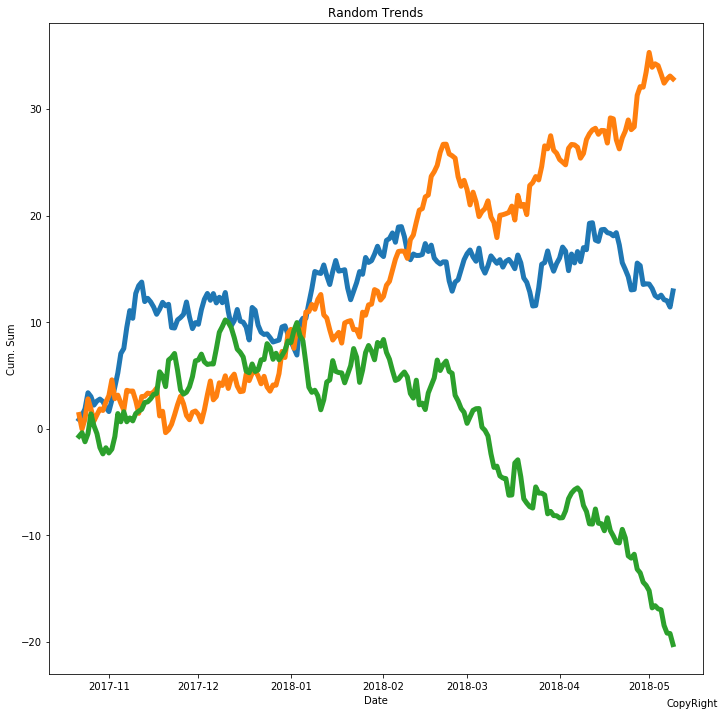<matplotlib.figure.Figure at 0x120e70828>

<matplotlib.figure.Figure at 0x120c16940>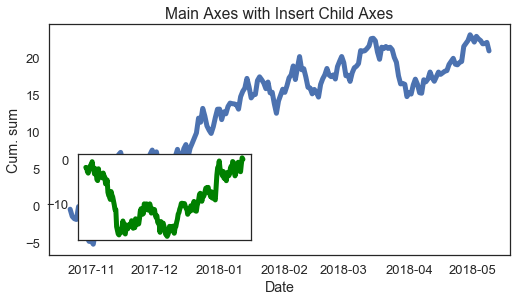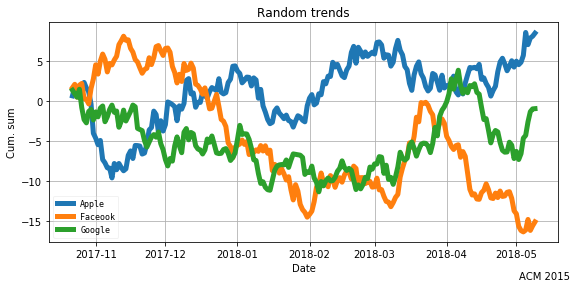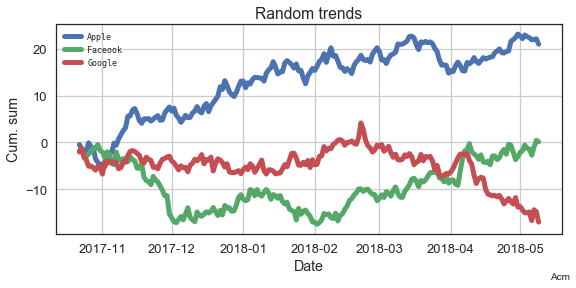<matplotlib.text.Text at 0x11eb71278>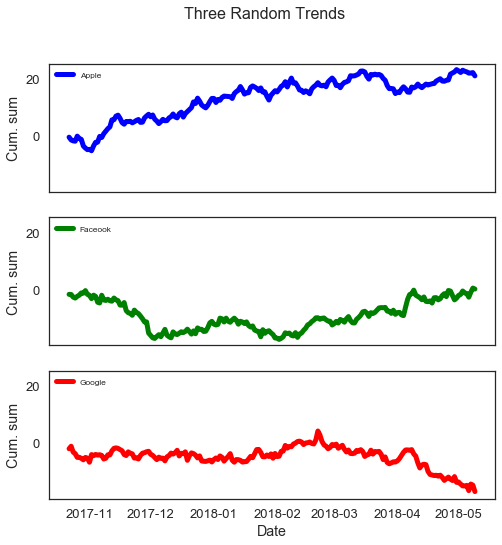常見圖表

1. 直方圖（Histogram）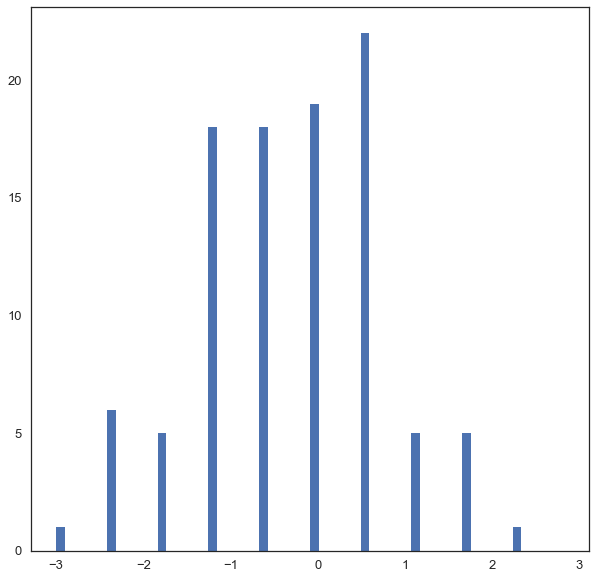2. 散佈圖（Scatter plot）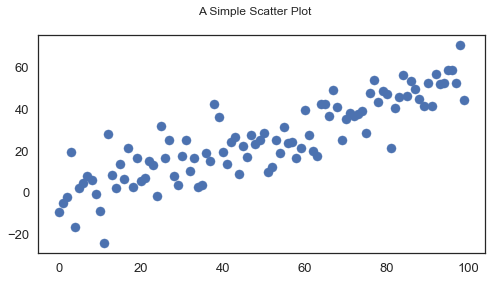3. 線圖（Line plot）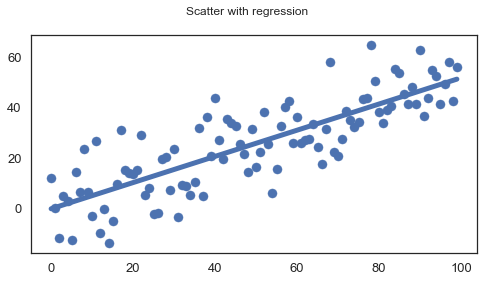4. 長條圖（Bar plot）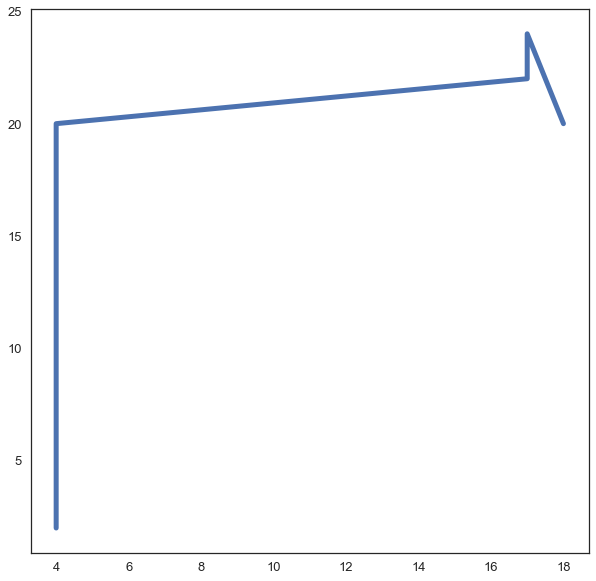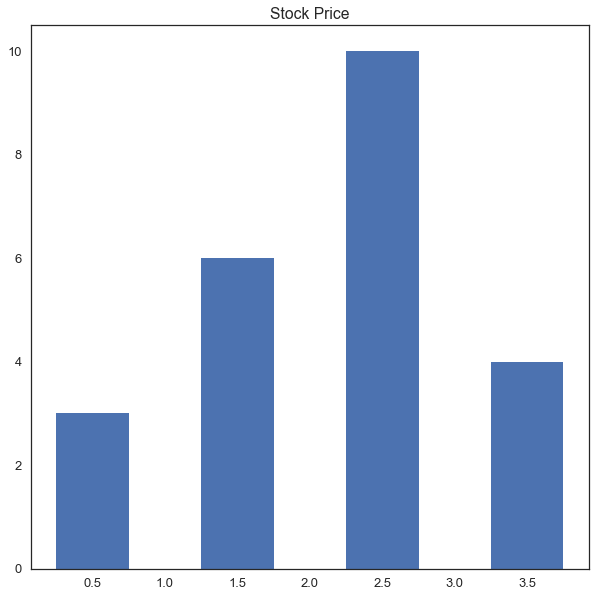5. 盒鬚圖（Box plot）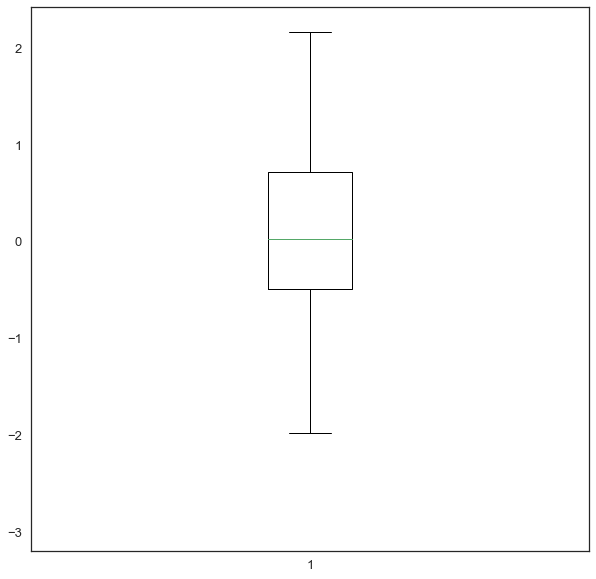6. 圓餅圖（Pie plot）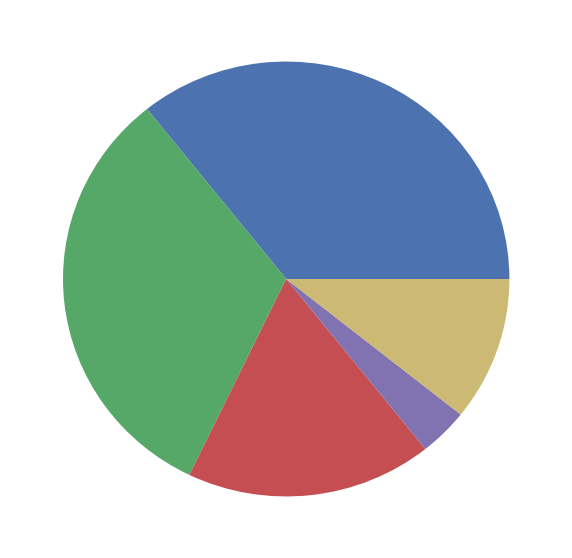Python 其他資料視覺化套件

1. Seaborn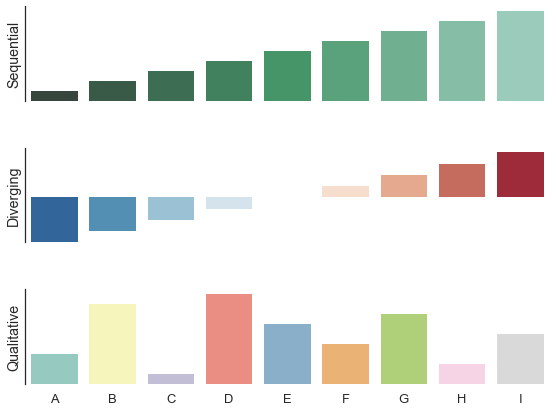2. Bokeh

延伸閱讀

（image via matplotlib

@kdchang 文藝型開發者，夢想是做出人們想用的產品和辦一所心目中理想的學校。A Starter & Maker. JavaScript, Python & Arduino/Android lover.:)